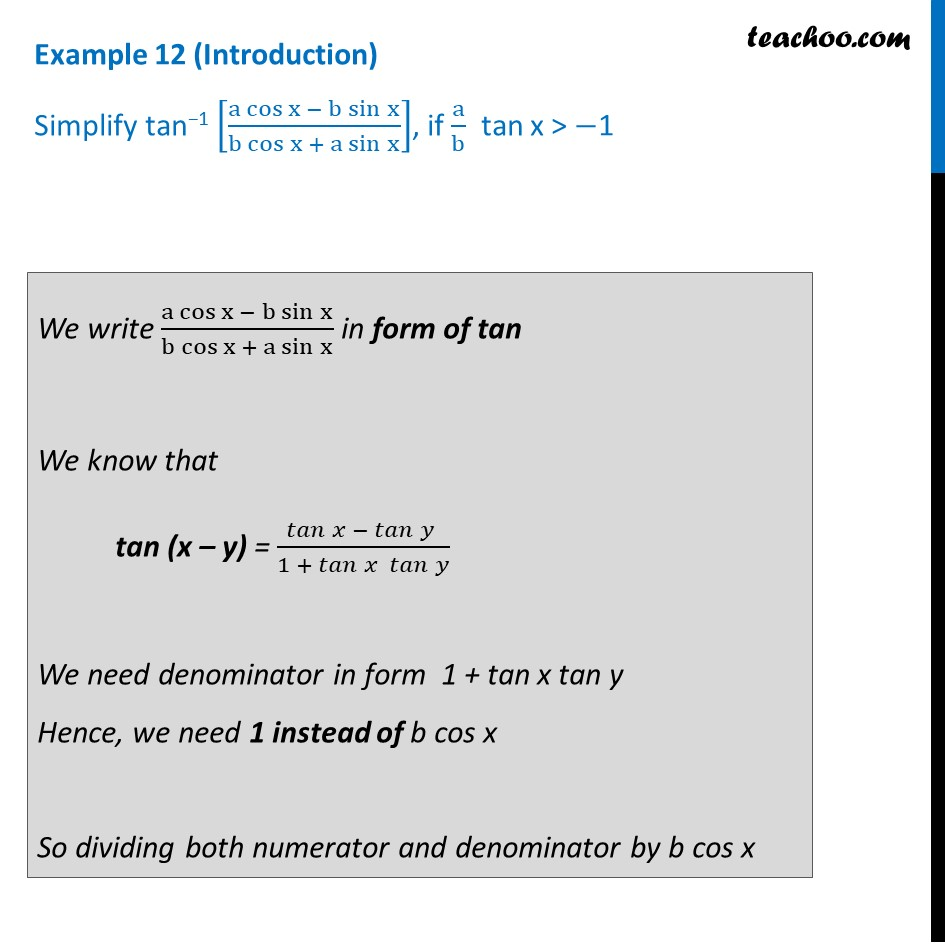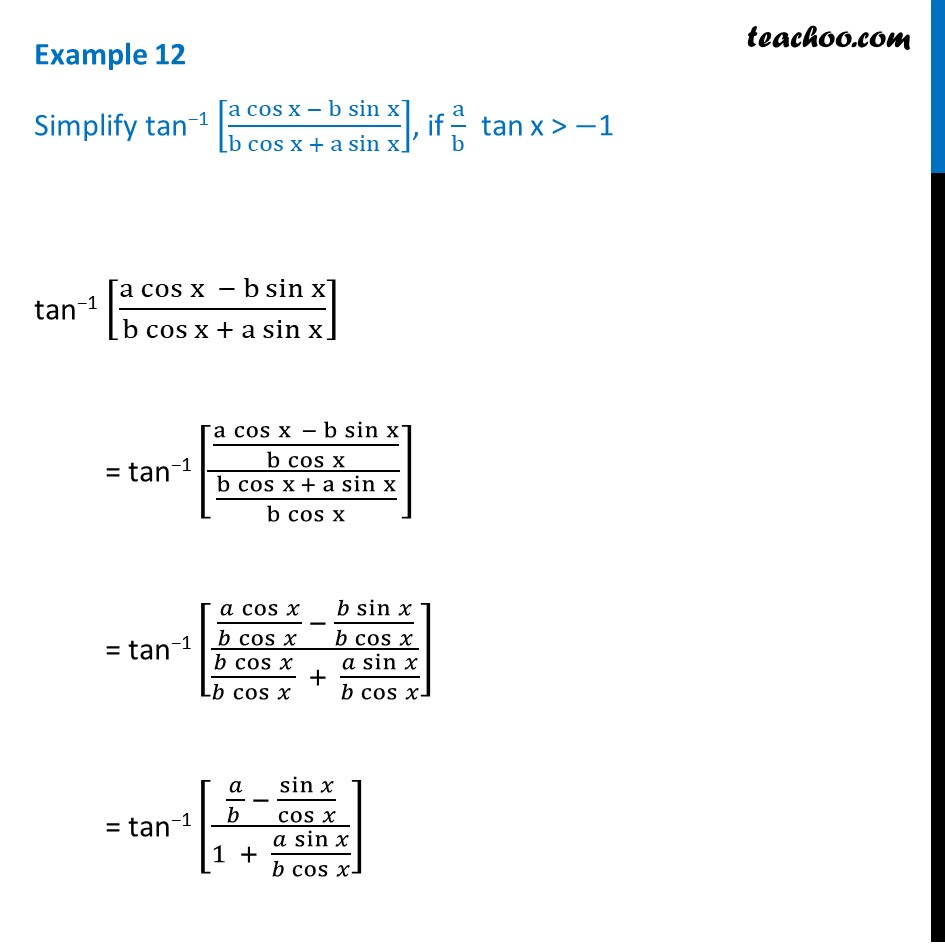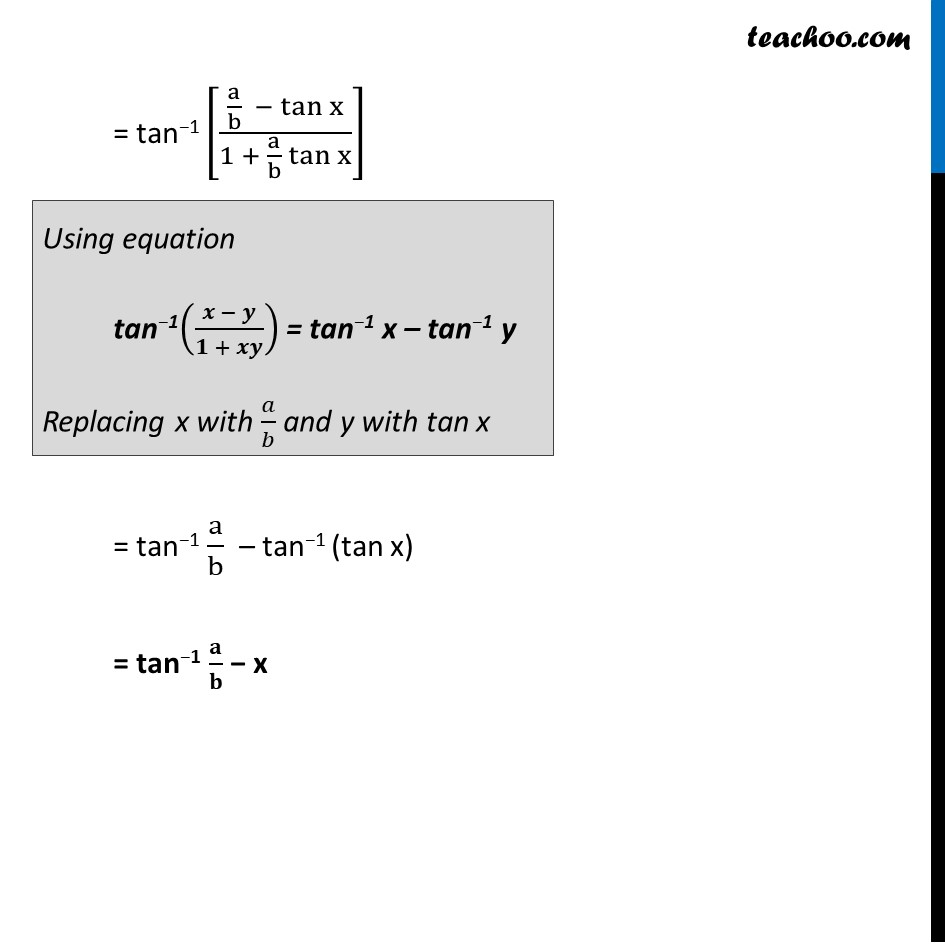Chapter 2 Class 12 Inverse Trigonometric Functions

Class 12
Important Questions for exams Class 12Learn in your speed, with individual attention - Teachoo Maths 1-on-1 Class

### Transcript

Question 6 (Introduction) Simplify tan−1 [(a cos⁡〖x − b sin⁡x 〗)/(b cos⁡〖x + a sin⁡x 〗 )], if a/b tan x > −1 We write (a cos⁡〖x − b sin⁡x 〗)/(b cos⁡〖x + a sin⁡x 〗 ) in form of tan We know that tan (x – y) = 𝑡𝑎𝑛⁡〖𝑥 −〖 𝑡𝑎𝑛〗⁡〖𝑦 〗 〗/(1 + 𝑡𝑎𝑛⁡〖𝑥 𝑡𝑎𝑛⁡𝑦 〗 ) We need denominator in form 1 + tan x tan y Hence, we need 1 instead of b cos x So dividing both numerator and denominator by b cos x Question 6 Simplify tan−1 [(a cos⁡〖x − b sin⁡x 〗)/(b cos⁡〖x + a sin⁡x 〗 )], if a/b tan x > −1 tan−1 [(a cos⁡〖x − b sin⁡x 〗)/(b cos⁡〖x + a sin⁡x 〗 )] = tan−1 [((a cos⁡〖x − b sin⁡x 〗)/(b cos⁡x ))/((b cos⁡〖x + a sin⁡x 〗)/(b cos⁡x ))] = tan−1 [((𝑎 cos⁡𝑥)/(𝑏 cos⁡𝑥 ) − (𝑏 sin⁡𝑥)/(𝑏 cos⁡𝑥 ))/((𝑏 cos⁡𝑥)/(𝑏 cos⁡𝑥 ) + (𝑎 sin⁡𝑥)/(𝑏 cos⁡𝑥 ))] = tan−1 [(𝑎/(𝑏 ) − sin⁡𝑥/cos⁡𝑥 )/(1 + (𝑎 sin⁡𝑥)/(𝑏 cos⁡𝑥 ))] = tan−1 [(a/b − tan⁡x)/(1 + a/b tan⁡x )] = tan−1 a/b – tan−1 (tan x) = tan−1 𝐚/𝐛 − x Using equation tan−1((𝒙 − 𝒚)/(𝟏 + 𝒙𝒚)) = tan−1 x – tan−1 y Replacing x with 𝑎/𝑏 and y with tan x